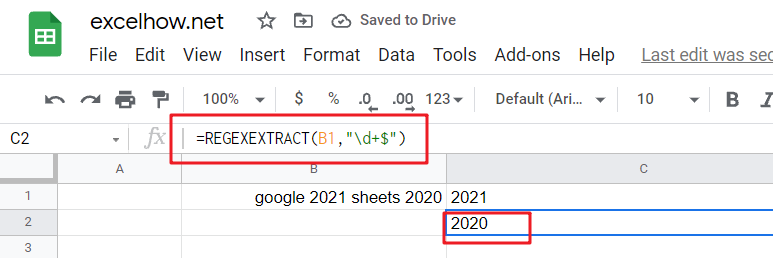# ExcelHow

This post will guide you how to use Google Sheets REGEXEXTRACT function with syntax and examples.

## Description

The Google Sheets REGEXEXTRACT function uses a regular expression to extract the first matching substring.

The REGEXEXTRACT function can be used to extract the first matching substring from a text string according to a given regular expression in google sheets. So it returns the part of the specified string that matches the given pattern in a regular expression.

The REGEXEXTRACT function is a build-in function in Google Sheets and it is categorized as a Text Function.

## Syntax

The syntax of the REGEXEXTRACT function is as below:

=REGEXEXTRACT (text, regular_expression)

Where the REGEXEXTRACT function arguments are:

• text -This is a required argument. The text string that you want to extract a substring based on regular expression.
• regular_expression – This is a required argument. The `Regular_expression` is a regular expression. It can be used to match the part of the text that you want to extract. The regular expression argument should be entered in double-quote characters.

Note:

• The REGEXEXTRACT function takes two arguments. It extracts the first matching substrings according to a given regular expression.
• The REGEXEXTRACT function also can return multiple results with capture groups. A capture group is also a part of a regular expression that can be enclosed in parentheses.
• The REGEXEXTRACT function only works with text value as its first argument and returns a text result. If you wish to return a numeric value, and you need to use VALUE function in combination with the REGEXEXTRACT function. If the input value is a number, and you can use the TEXT function to convert them.
• Google Sheets supports RE2 for regular expressions.

## Google Sheets REGEXEXTRACT Function Examples

The below examples will show you how to use google sheets REGEXEXTRACT function to extract a substring from a text according to a regular expression.

#1 Extract the first 5 characters from a string using REGEXEXTRACT function with a regular expression “…..” , type:

`=REGEXEXTRACT(B1,".....") //it returns “googl”`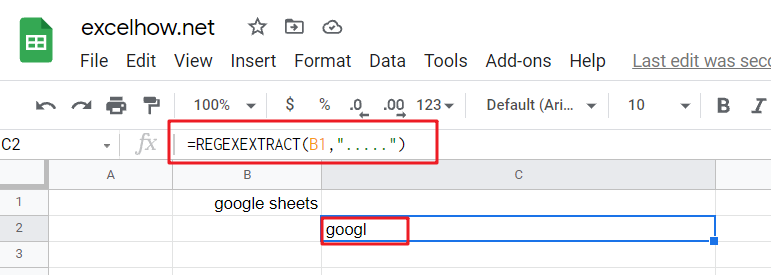#2 Extract the last 5 characters from a string using REGEXEXTRACT function with a regular expression “…..\$” , type:

`=REGEXEXTRACT(B1,".....\$") // it returns “heets”`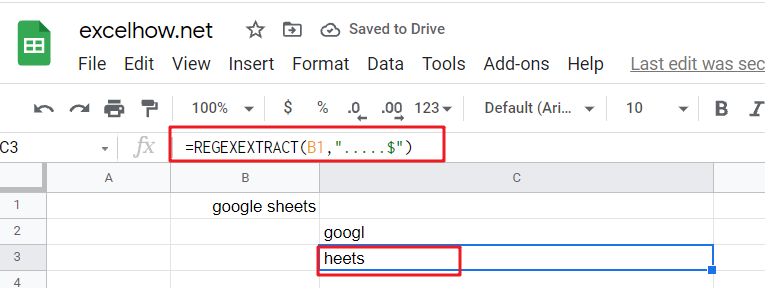#3 Extract the first whole word from a string using REGEXEXTRACT function with a regular expression “\w+” , type:

`=REGEXEXTRACT(B1,"\w+") // it returns “google”`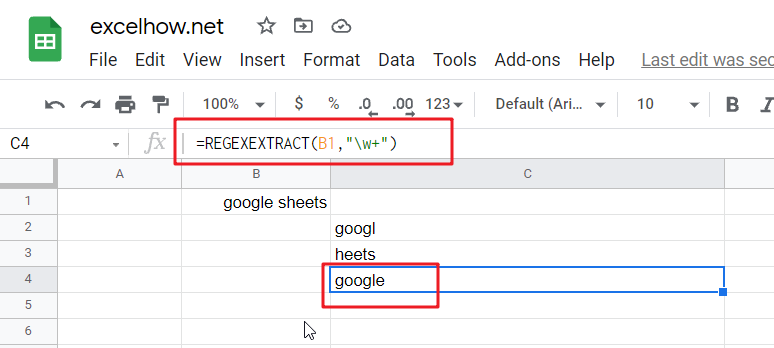#4 Extract the last whole word from a string using REGEXEXTRACT function with a regular expression “\w+\$” , type:

`=REGEXEXTRACT(B1,"\w+\$") // it returns “sheets”`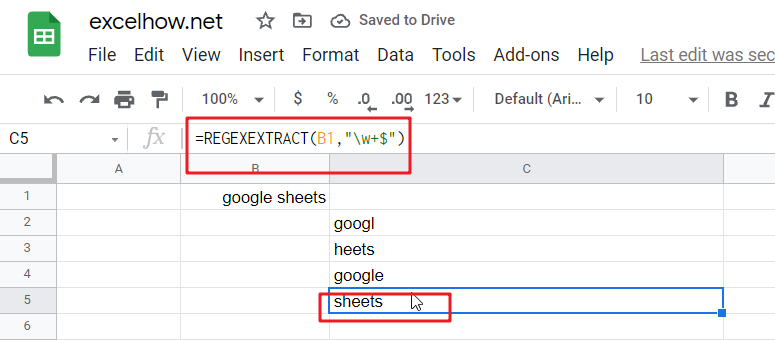#5 Extract the first number from a string using REGEXEXTRACT function with a regular expression “\d+” , type:

`=REGEXEXTRACT(B1,"\d+") // it returns “2021”`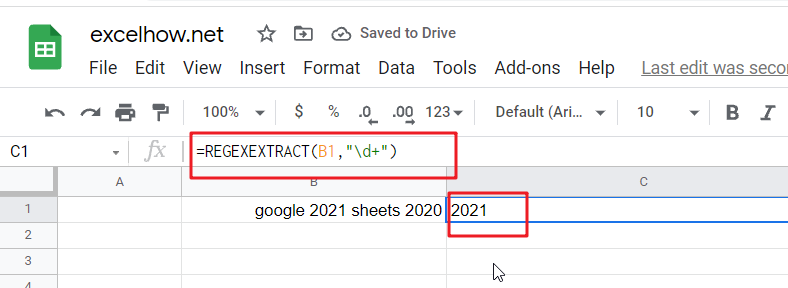#6 Extract the last number from a string using REGEXEXTRACT function with a regular expression “\d+\$” , type:

`=REGEXEXTRACT(B1,"\d+") // it returns “2020”`